# RD Sharma Solutions for Class 10 Maths Chapter 14- Co-ordinate Geometry Exercise 14.2

After getting to know how to locate points and write their coordinates, the next step would be to find the distance between two points. In this exercise, students will be solving problems based on finding the distance between two points. Using this concept, students will have to prove the properties of a given figure and also prove the collinearity of three points. The RD Sharma Solutions Class 10 is a valuable resource for students to resolve problems that come up in their class. Also, download the RD Sharma Solutions for Class 10 Maths Chapter 14 Co-ordinate Geometry Exercise 14.2, PDF given below.

## RD Sharma Solutions for Class 10 Maths Chapter 14 Co-ordinate Geometry Exercise 14.2 Download PDF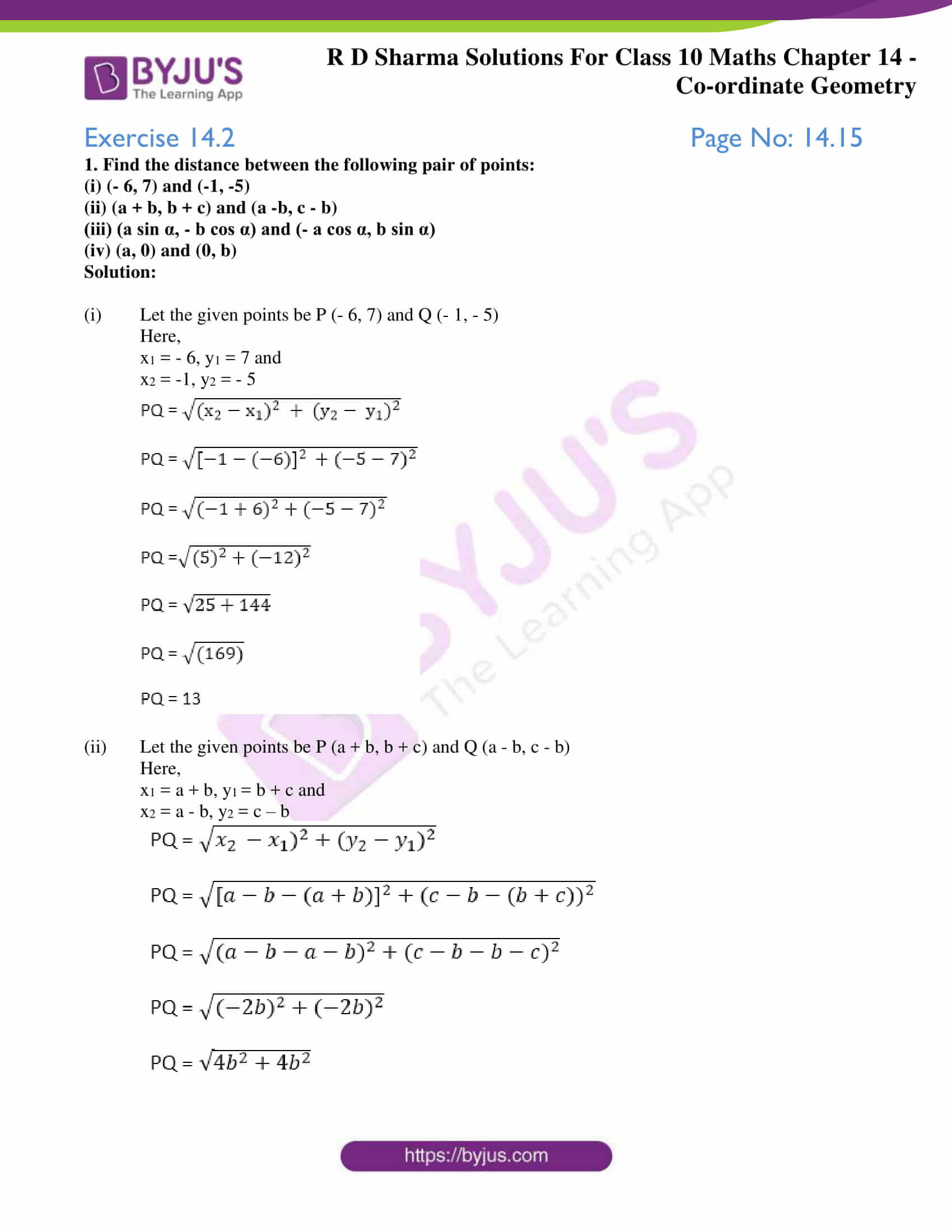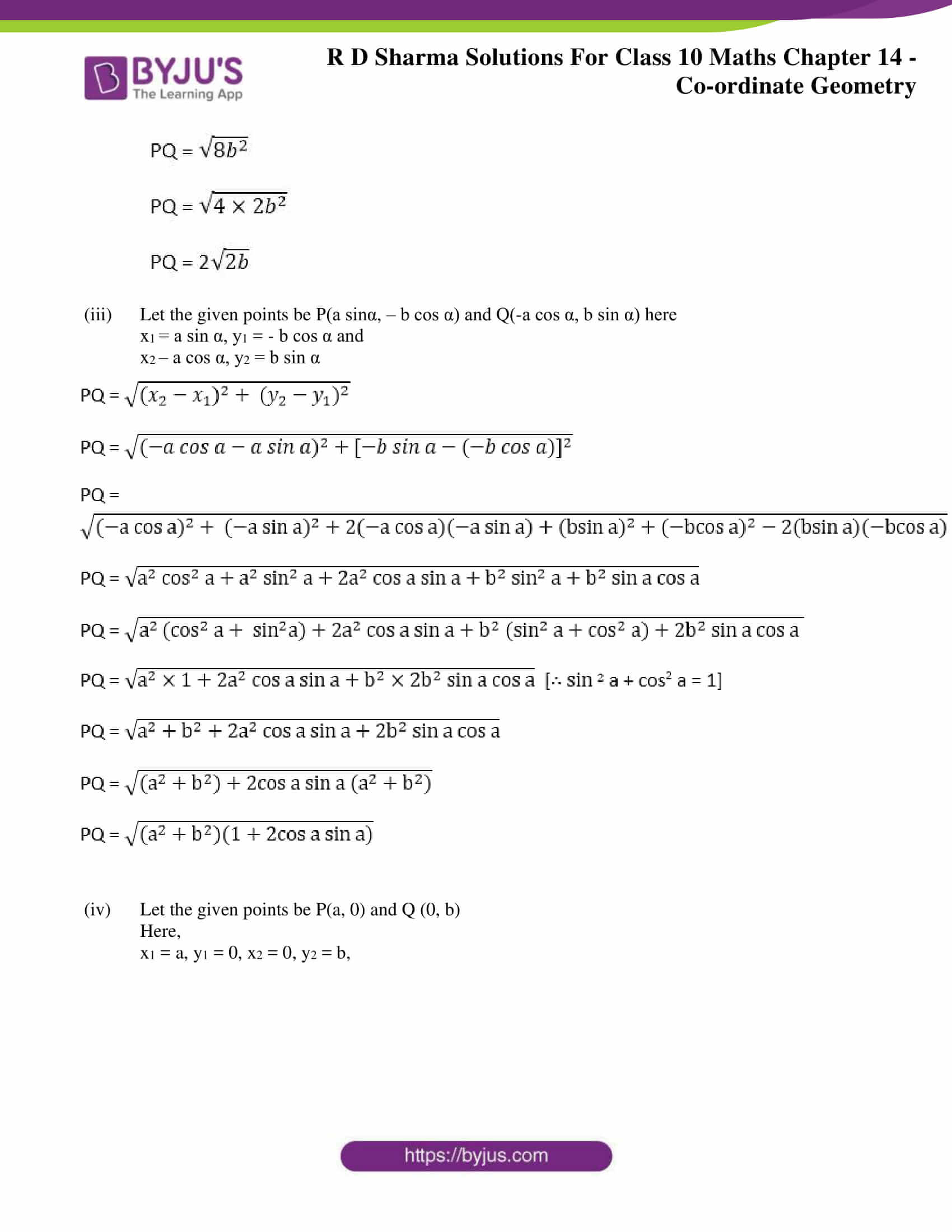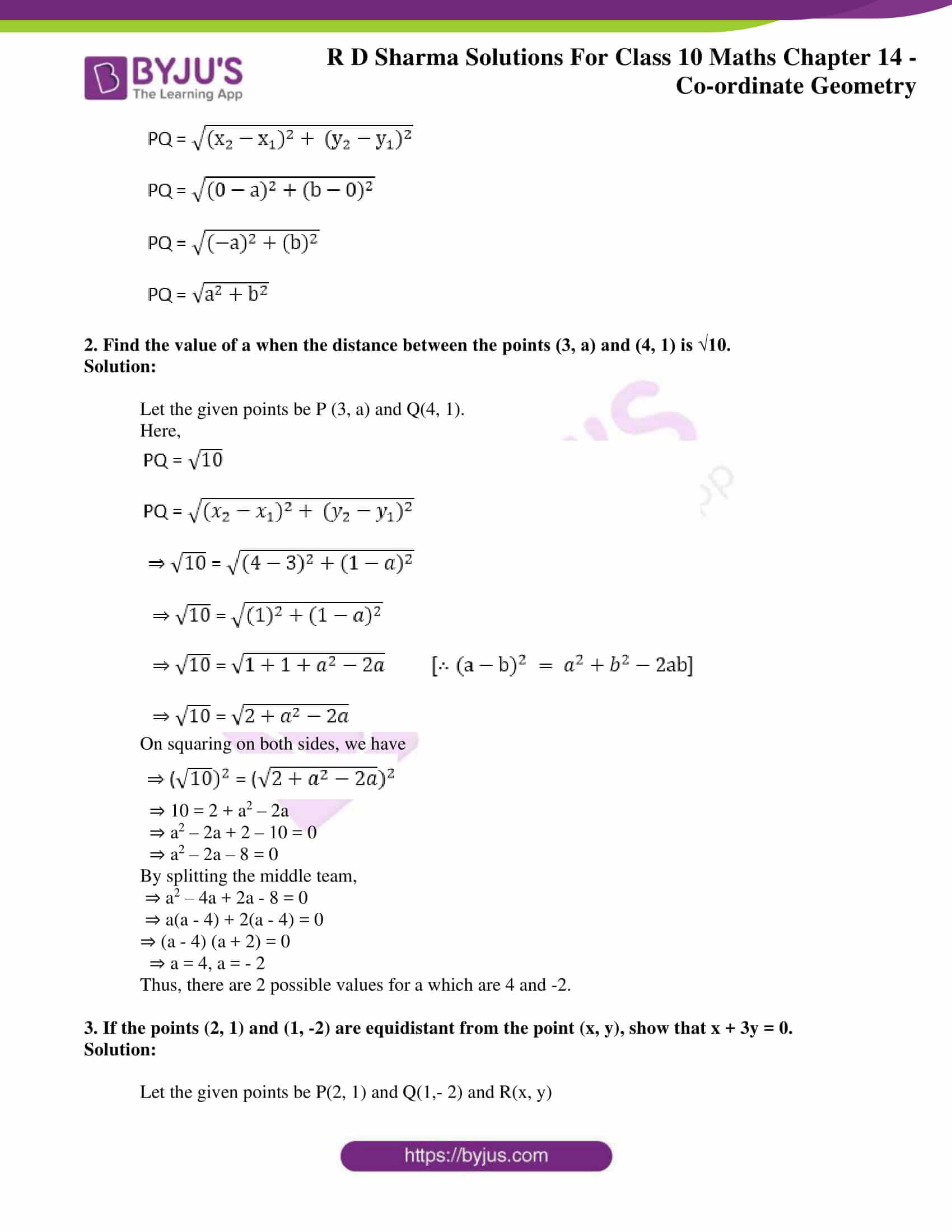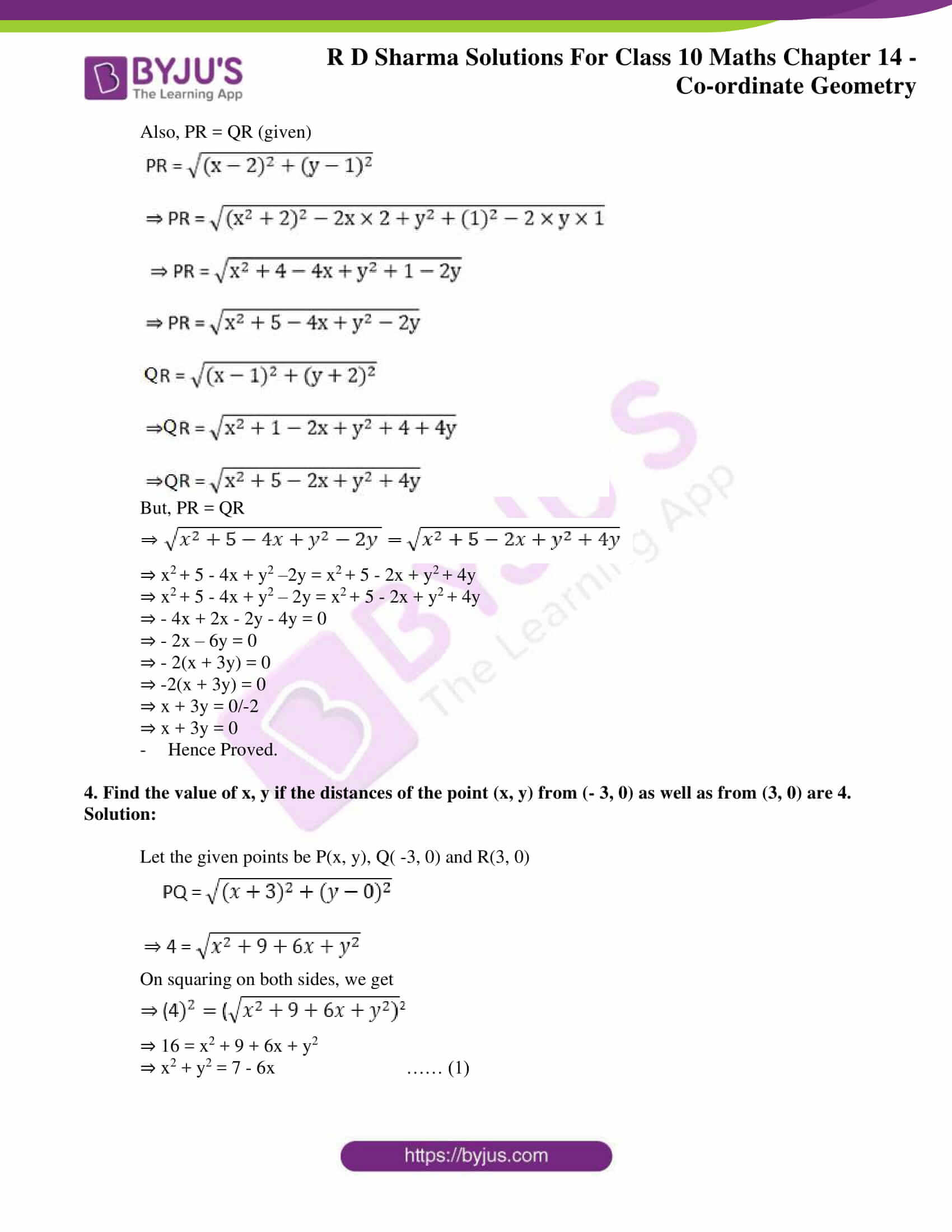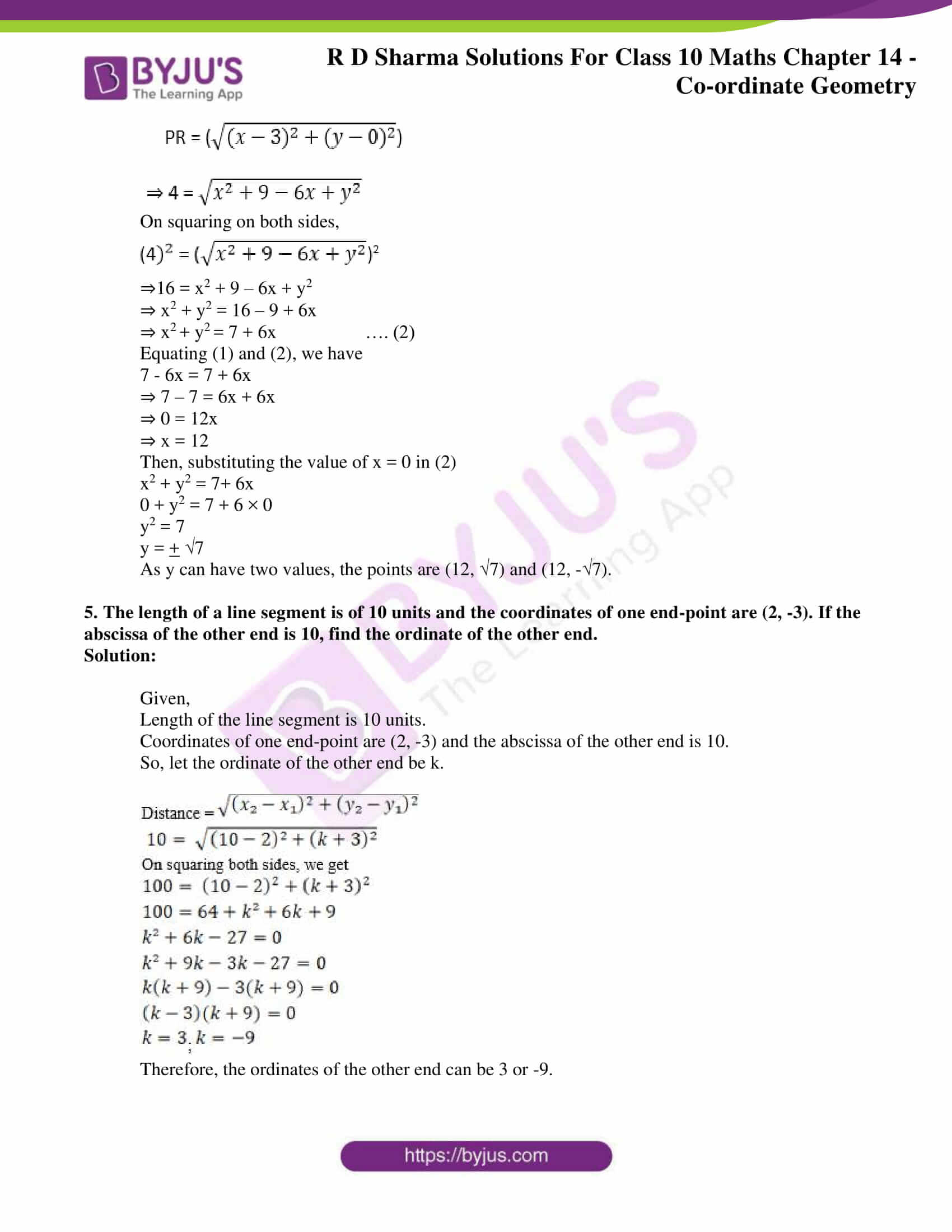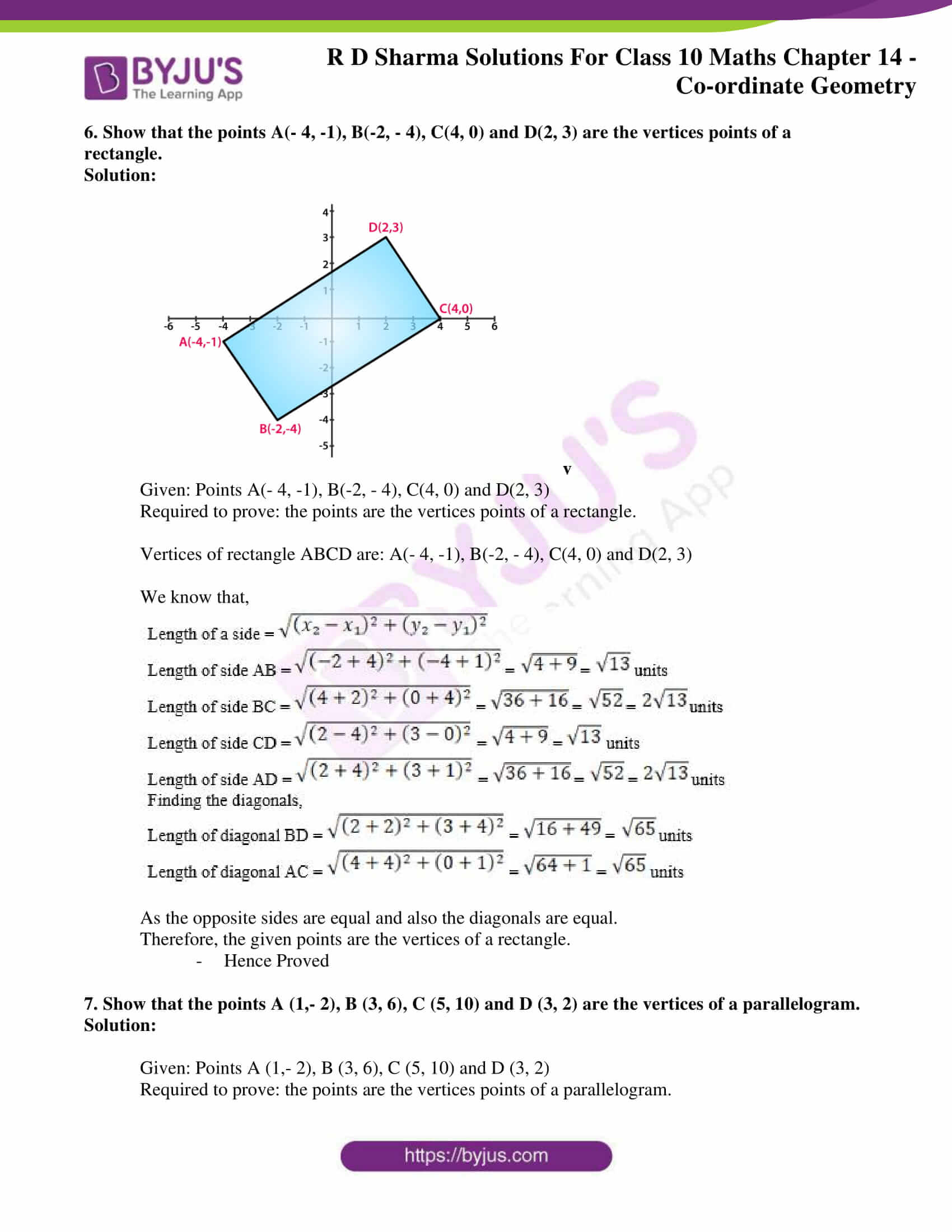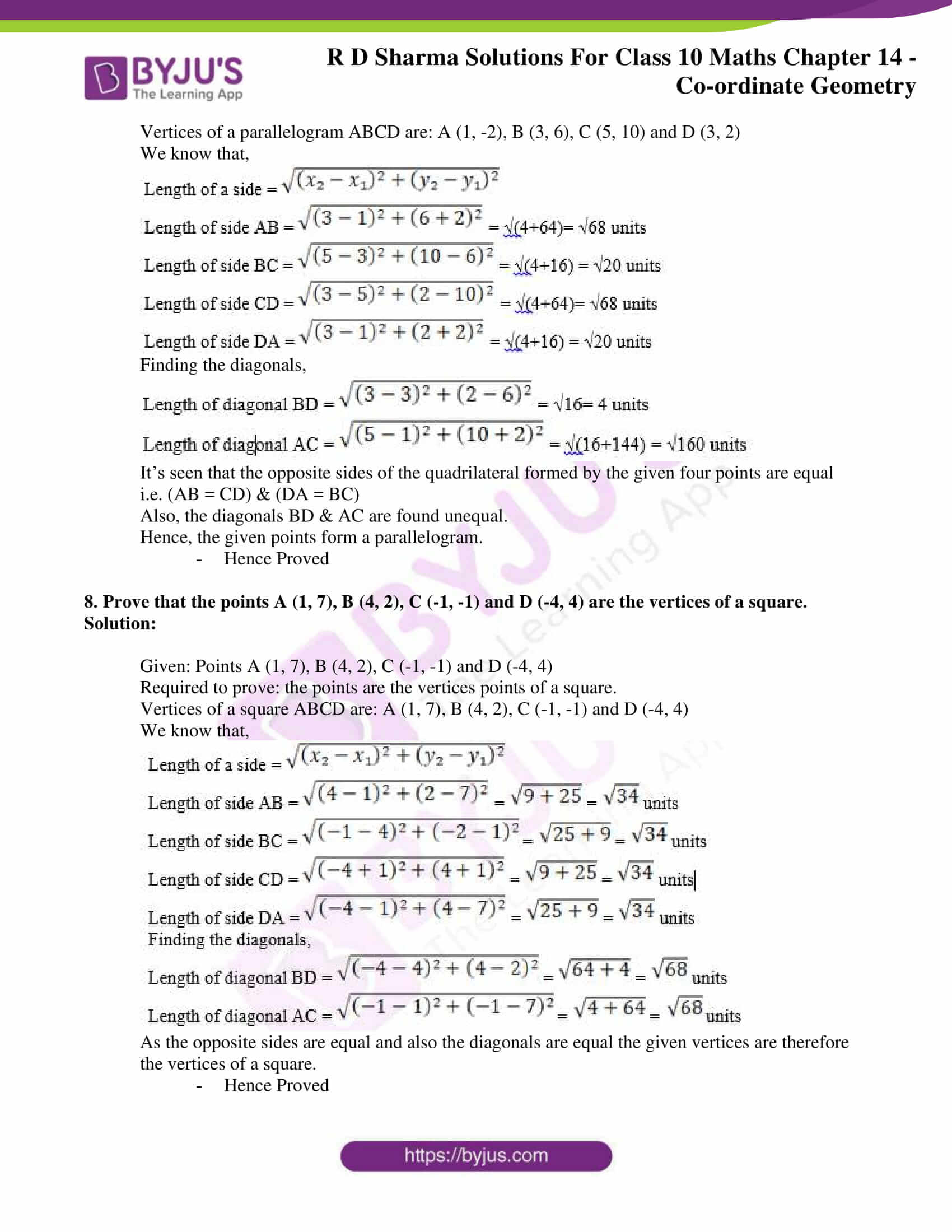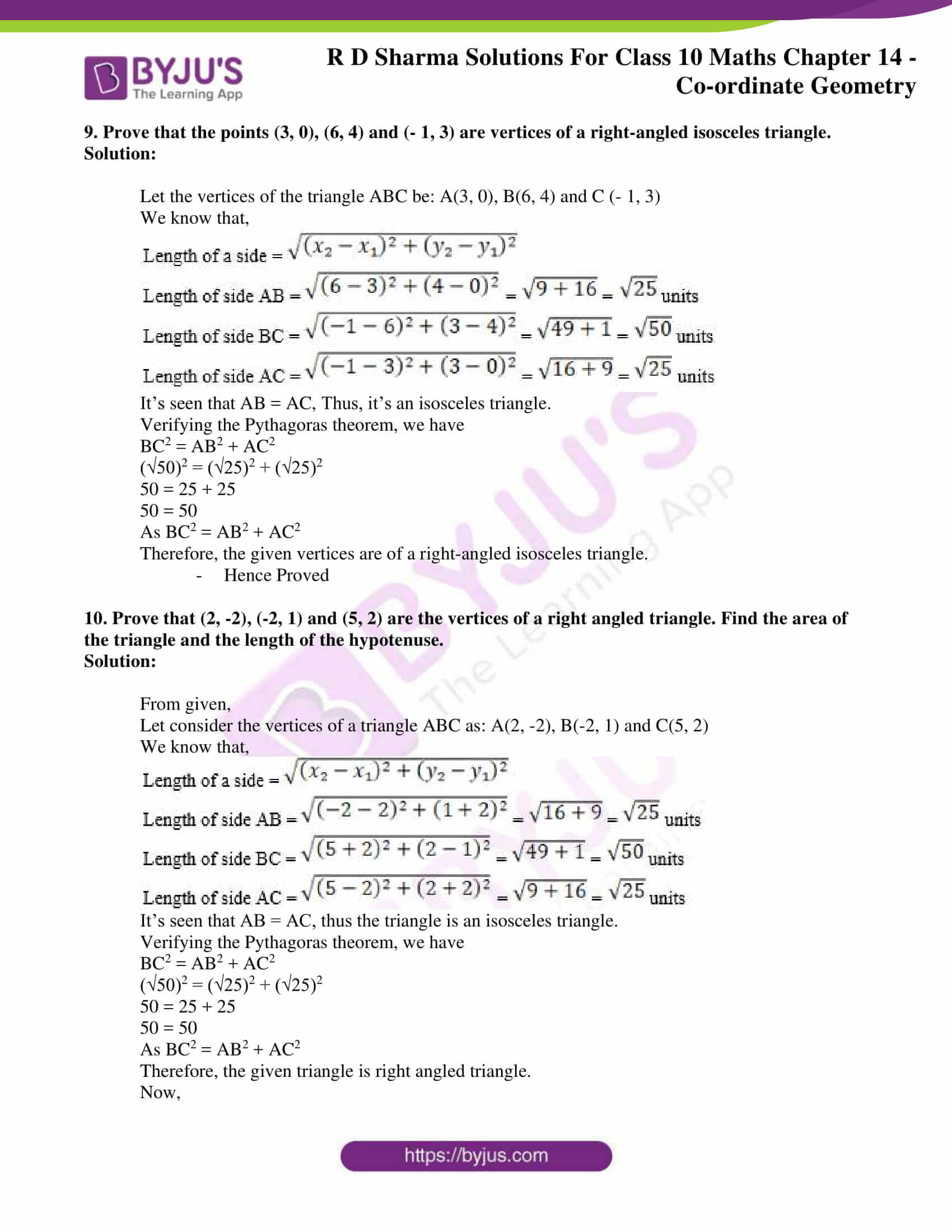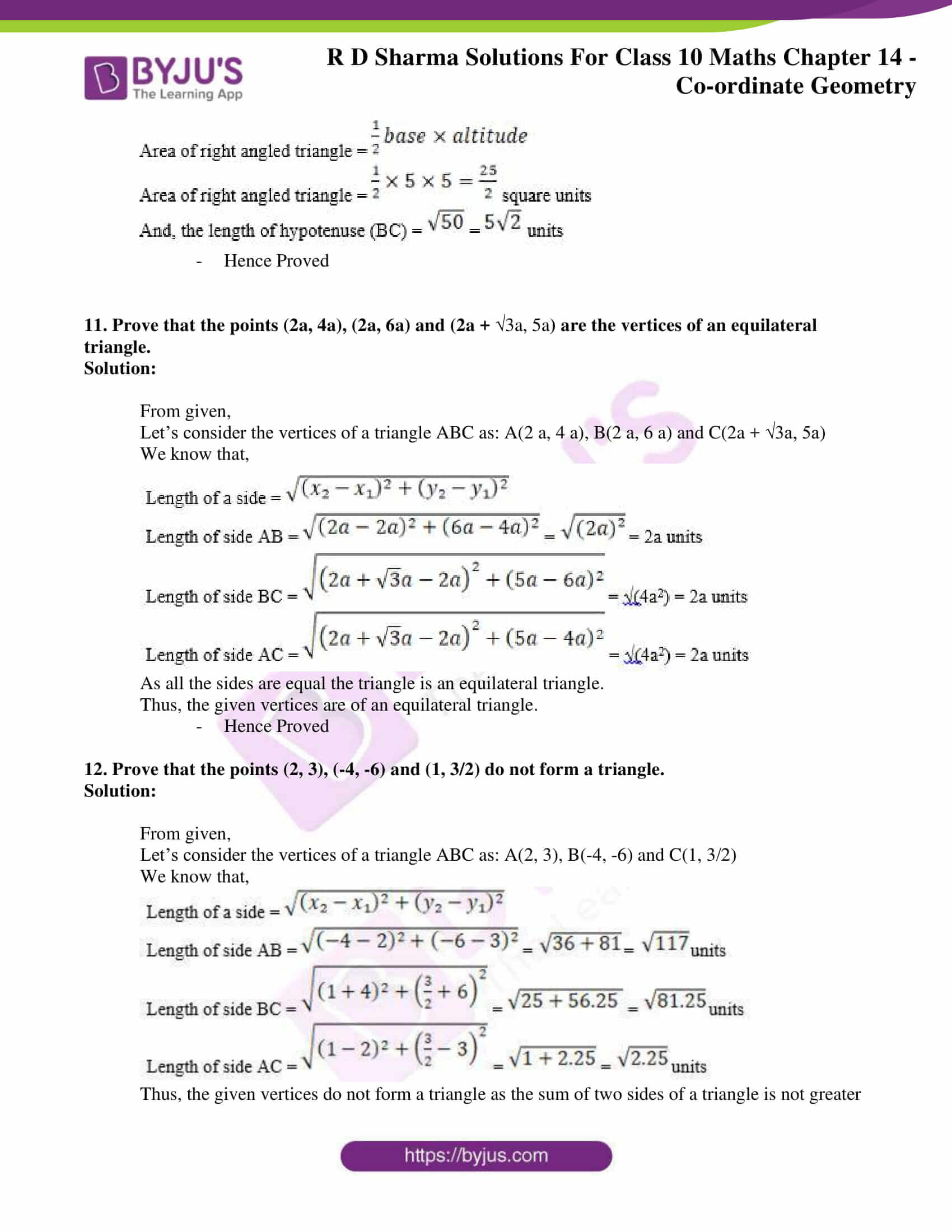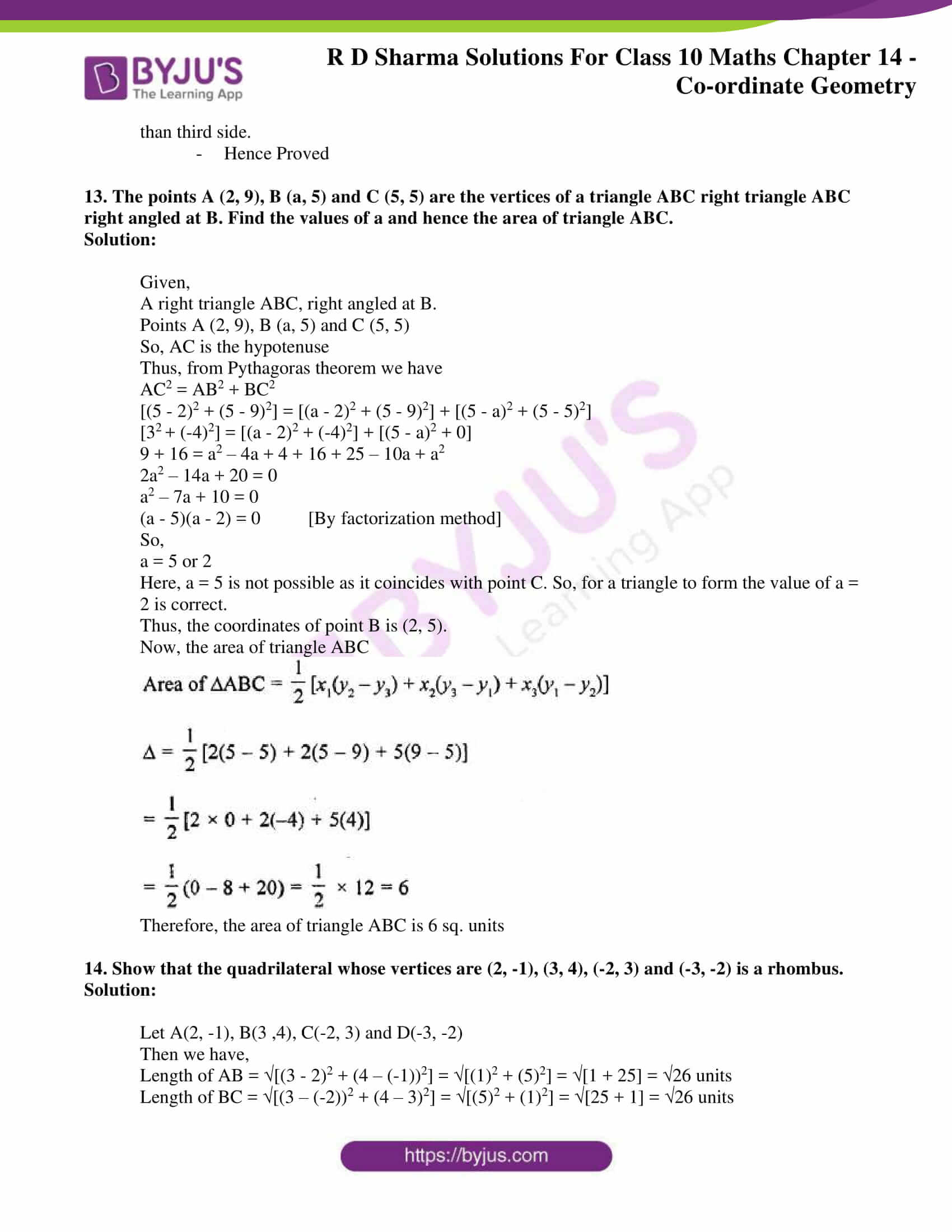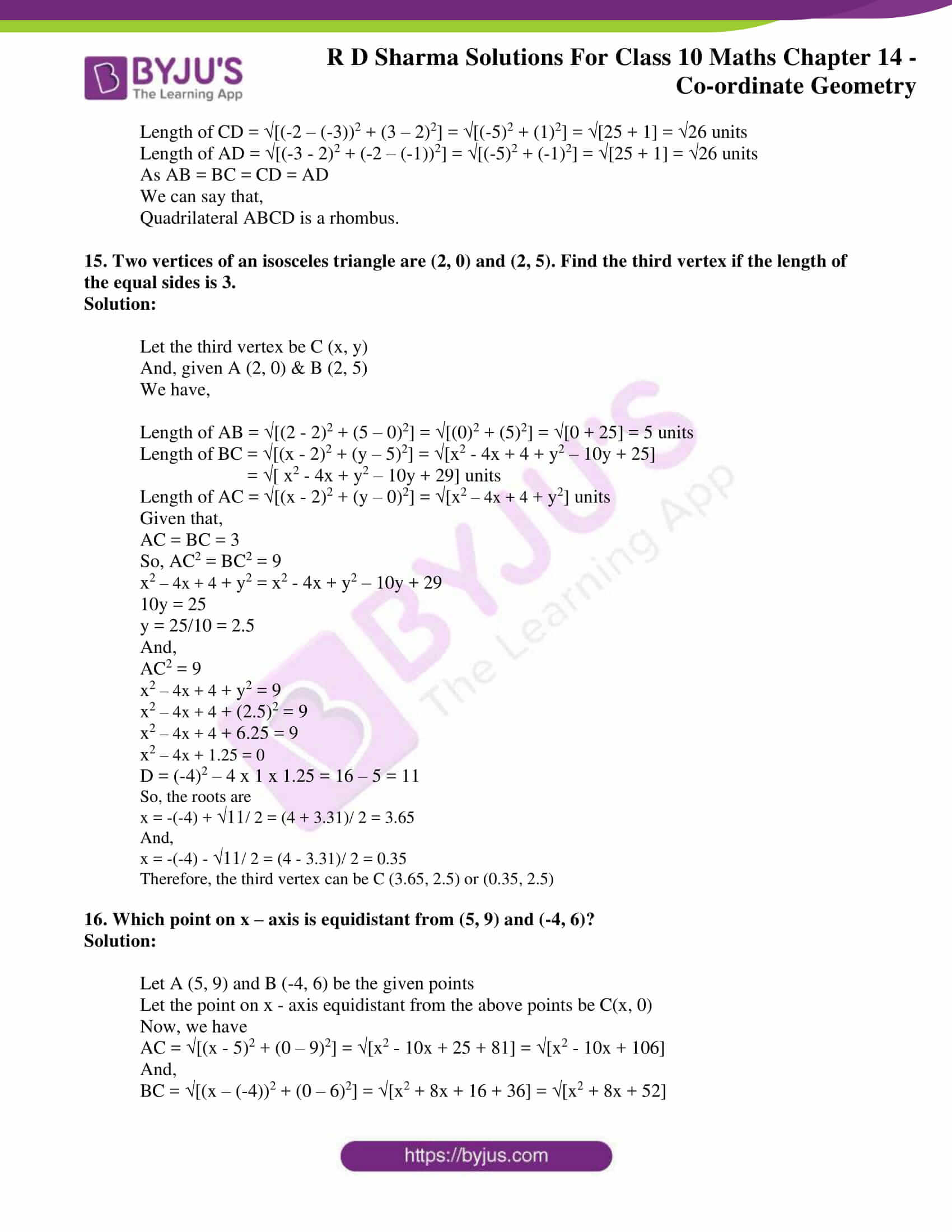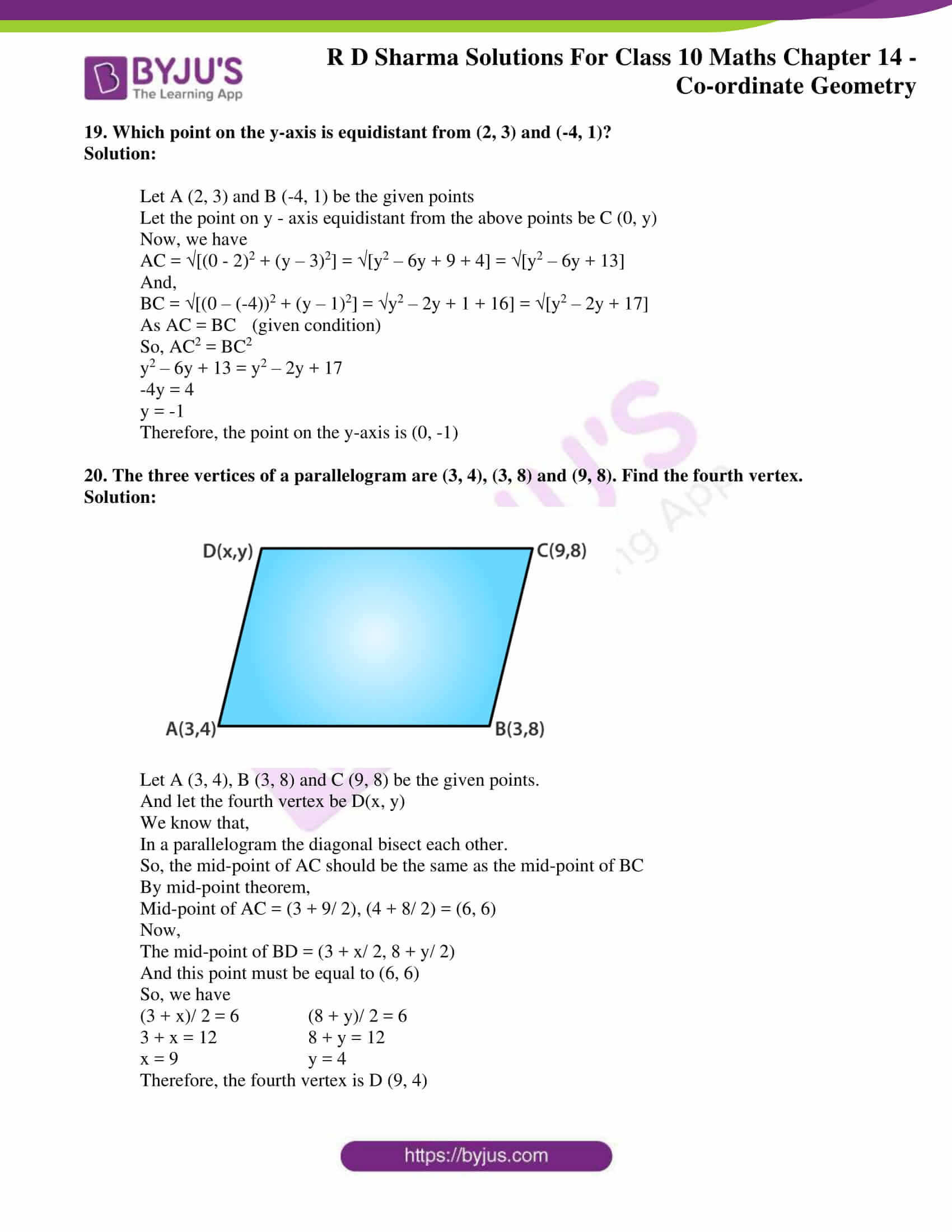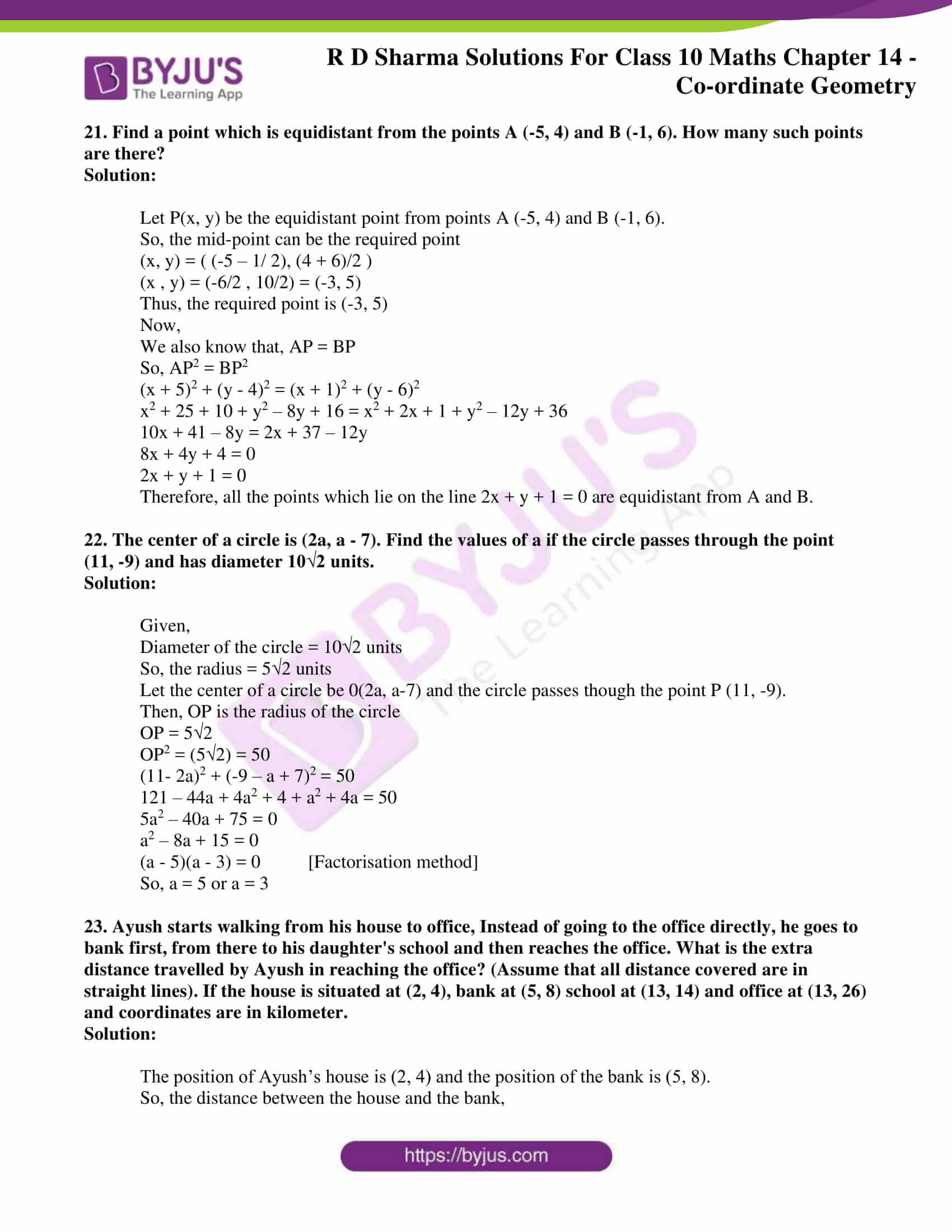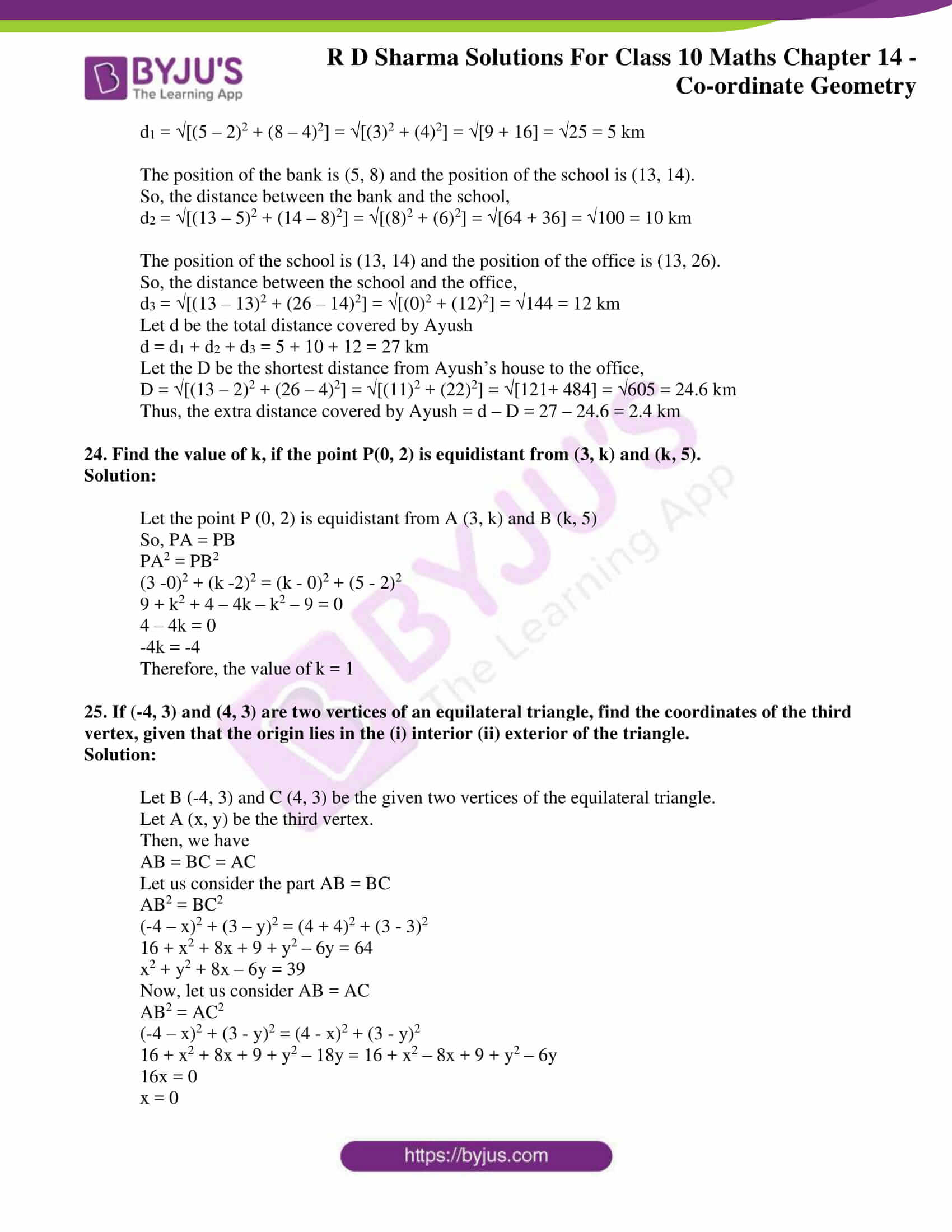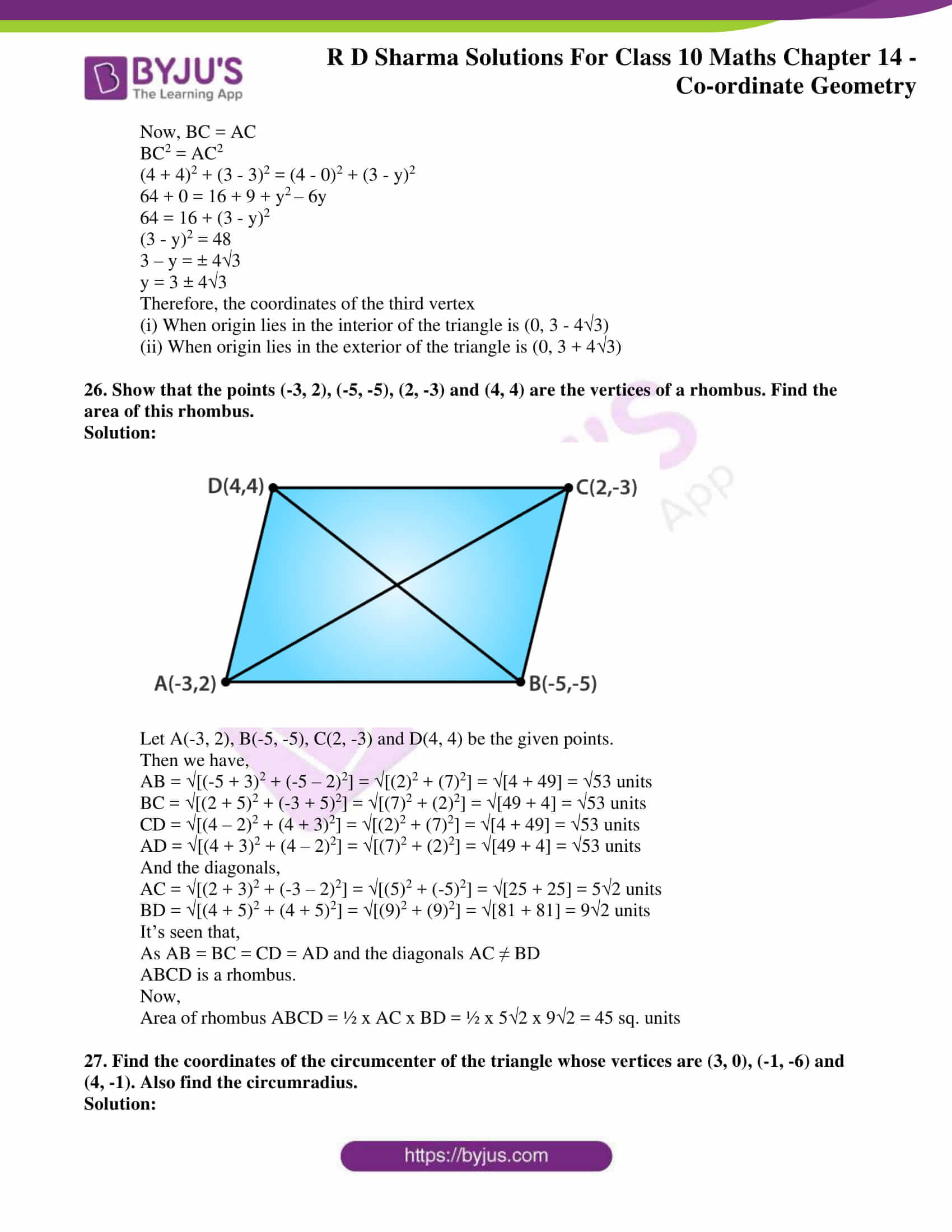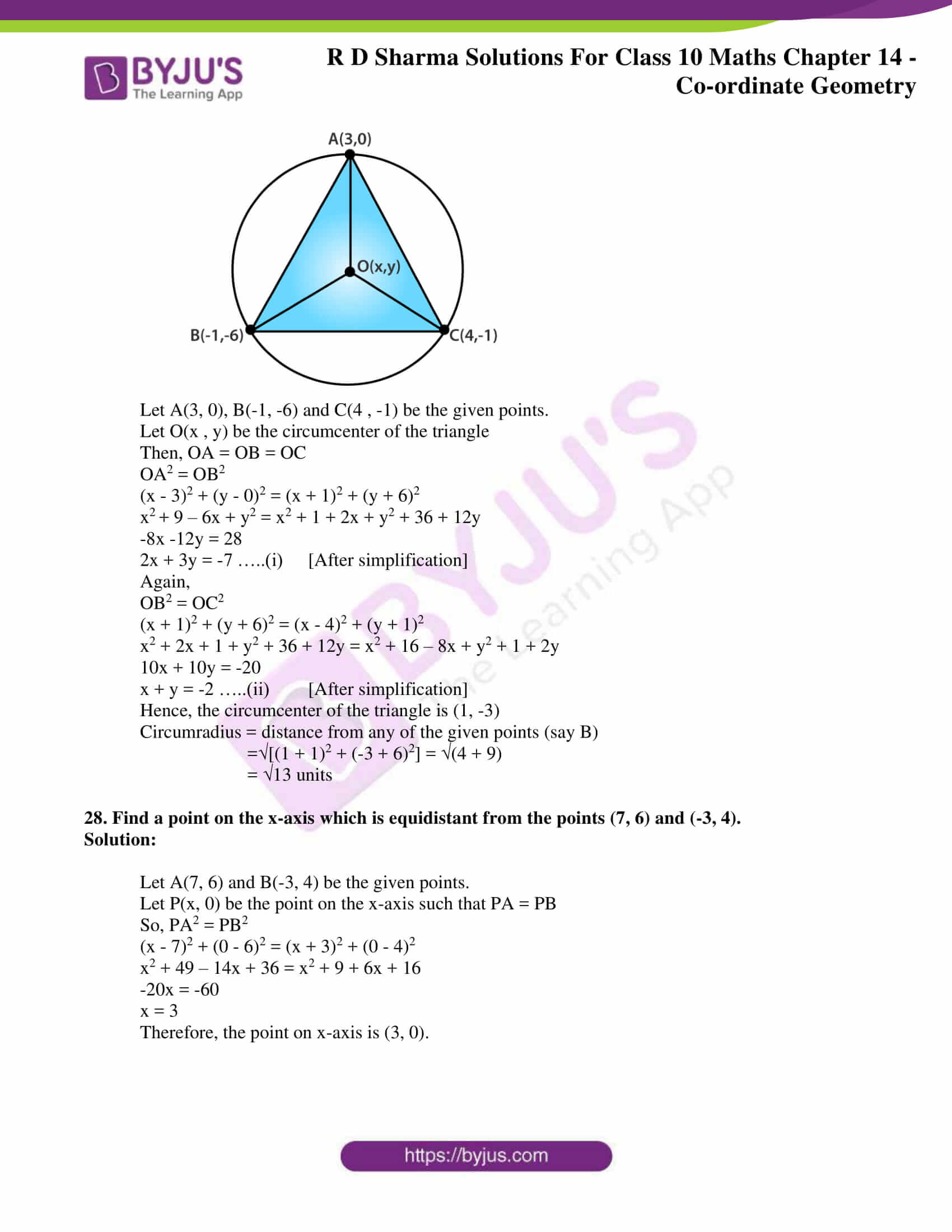### Access RD Sharma Solutions for Class 10 Maths Chapter 14 Co-ordinate Geometry Exercise 14.2

1. Find the distance between the following pair of points:

(i) (- 6, 7) and (-1, -5)

(ii) (a + b, b + c) and (a -b, c – b)

(iii) (a sin α, – b cos α) and (- a cos α, b sin α)

(iv) (a, 0) and (0, b)

Solution:

(i) Let the given points be P (- 6, 7) and Q (- 1, – 5)

Here,

x1 = – 6, y1 = 7 and

x2 = -1, y2 = – 5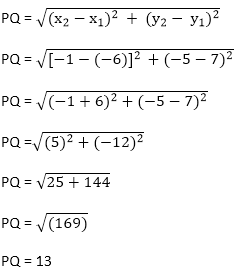(ii) Let the given points be P (a + b, b + c) and Q (a – b, c – b)

Here,

x1 = a + b, y= b + c and

x2 = a – b, y2 = c – b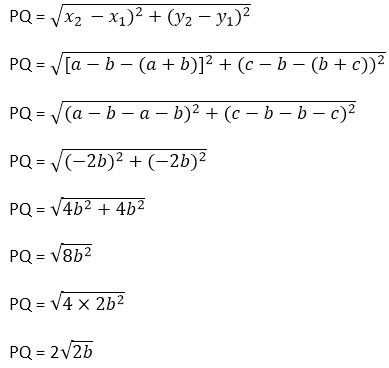(iii) Let the given points be P(a sinα, – b cos α) and Q(-a cos α, b sin α) here

x= a sin α, y1 = – b cos α and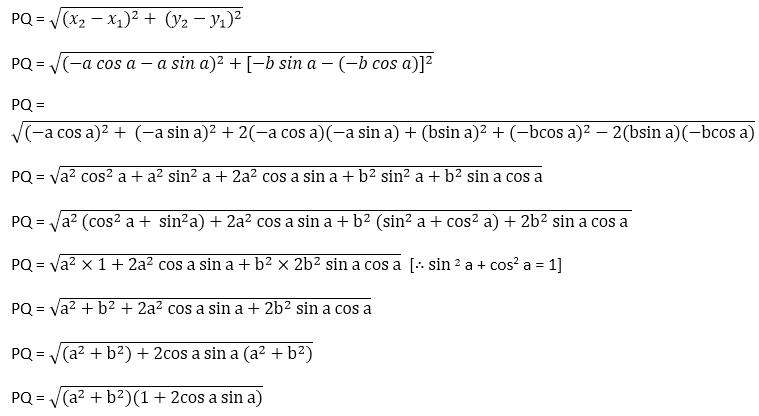x– a cos α, y2 = b sin α

(iv) Let the given points be P(a, 0) and Q (0, b)

Here,

x1 = a, y1 = 0, x2 = 0, y2 = b,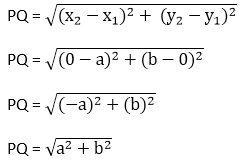2. Find the value of a when the distance between the points (3, a) and (4, 1) is √10.

Solution:

Let the given points be P (3, a) and Q(4, 1).

Here,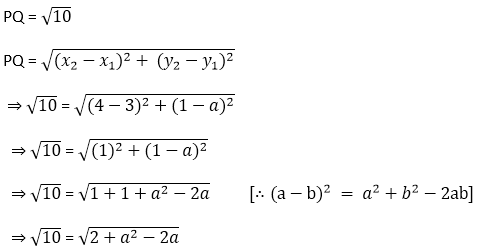On squaring on both sides, we have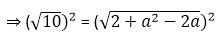⇒ 10 = 2 + a2 – 2a

⇒ a2 – 2a + 2 – 10 = 0

⇒ a2 – 2a – 8 = 0

By splitting the middle team,

⇒ a2 – 4a + 2a – 8 = 0

⇒ a(a – 4) + 2(a – 4) = 0

⇒ (a – 4) (a + 2) = 0

⇒ a = 4, a = – 2

Thus, there are 2 possible values for a which are 4 and -2.

3. If the points (2, 1) and (1, -2) are equidistant from the point (x, y), show that x + 3y = 0.

Solution:

Let the given points be P(2, 1) and Q(1,- 2) and R(x, y)

Also, PR = QR (given)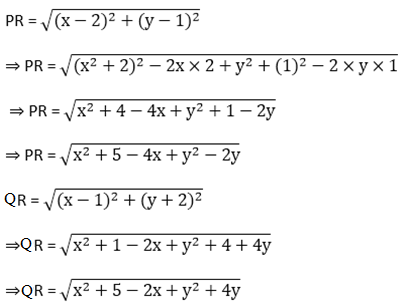But, PR = QR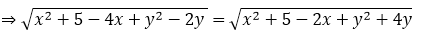⇒ x+ 5 – 4x + y2 –2y = x+ 5 – 2x + y+ 4y

⇒ x+ 5 – 4x + y2 – 2y = x+ 5 – 2x + y+ 4y

⇒ – 4x + 2x – 2y – 4y = 0

⇒ – 2x – 6y = 0

⇒ – 2(x + 3y) = 0

⇒ -2(x + 3y) = 0

⇒ x + 3y = 0/-2

⇒ x + 3y = 0

• Hence Proved.

4. Find the value of x, y if the distances of the point (x, y) from (- 3, 0) as well as from (3, 0) are 4.

Solution:

Let the given points be P(x, y), Q( -3, 0) and R(3, 0)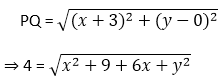On squaring on both sides, we get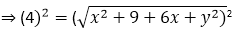⇒ 16 = x2 + 9 + 6x + y2

⇒ x2 + y2 = 7 – 6x                            …… (1)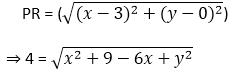On squaring on both sides,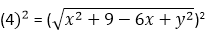⇒16 = x2 + 9 – 6x + y2

⇒ x2 + y2 = 16 – 9 + 6x

⇒ x2 + y= 7 + 6x                   …. (2)

Equating (1) and (2), we have

7 – 6x = 7 + 6x

⇒ 7 – 7 = 6x + 6x

⇒ 0 = 12x

⇒ x = 12

Then, substituting the value of x = 0 in (2)

x2 + y2 = 7+ 6x

0 + y2 = 7 + 6 × 0

y2 = 7

y = + √7

As y can have two values, the points are (12, √7) and (12, -√7).

5. The length of a line segment is of 10 units and the coordinates of one end-point are (2, -3). If the abscissa of the other end is 10, find the ordinate of the other end.

Solution:

Given,

Length of the line segment is 10 units.

Coordinates of one end-point are (2, -3) and the abscissa of the other end is 10.

So, let the ordinate of the other end be k.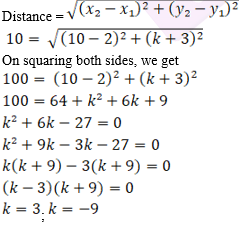Therefore, the ordinates of the other end can be 3 or -9.

6. Show that the points A(- 4, -1), B(-2, – 4), C(4, 0) and D(2, 3) are the vertices points of a rectangle.

Solution: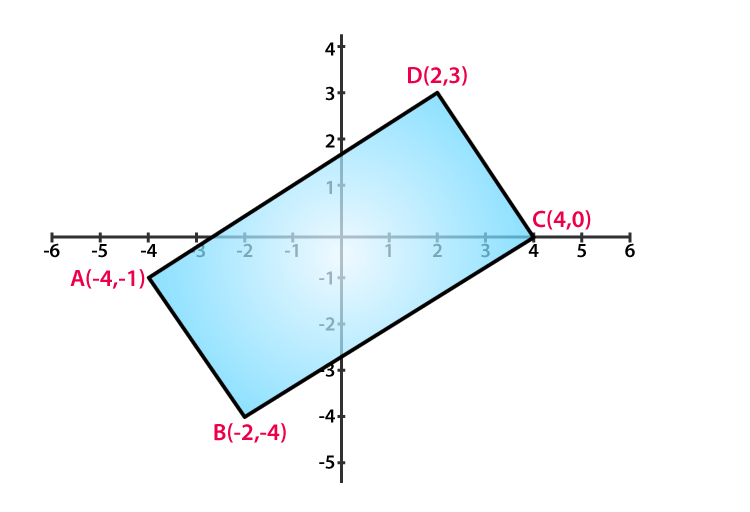v

Given: Points A(- 4, -1), B(-2, – 4), C(4, 0) and D(2, 3)

Required to prove: the points are the vertices points of a rectangle.

Vertices of rectangle ABCD are: A(- 4, -1), B(-2, – 4), C(4, 0) and D(2, 3)

We know that,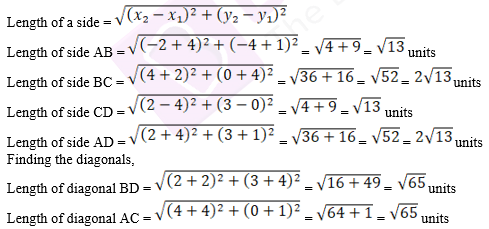As the opposite sides are equal and also the diagonals are equal.

Therefore, the given points are the vertices of a rectangle.

• Hence Proved

7. Show that the points A (1,- 2), B (3, 6), C (5, 10) and D (3, 2) are the vertices of a parallelogram.

Solution:

Given: Points A (1,- 2), B (3, 6), C (5, 10) and D (3, 2)

Required to prove: the points are the vertices points of a parallelogram.

Vertices of a parallelogram ABCD are: A (1, -2), B (3, 6), C (5, 10) and D (3, 2)

We know that,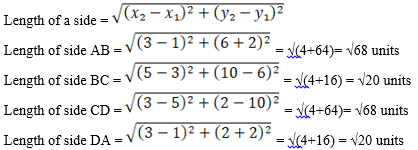Finding the diagonals,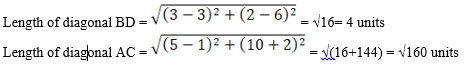It’s seen that the opposite sides of the quadrilateral formed by the given four points are equal

i.e. (AB = CD) & (DA = BC)

Also, the diagonals BD & AC are found unequal.

Hence, the given points form a parallelogram.

• Hence Proved

8. Prove that the points A (1, 7), B (4, 2), C (-1, -1) and D (-4, 4) are the vertices of a square.

Solution:

Given: Points A (1, 7), B (4, 2), C (-1, -1) and D (-4, 4)

Required to prove: the points are the vertices points of a square.

Vertices of a square ABCD are: A (1, 7), B (4, 2), C (-1, -1) and D (-4, 4)

We know that,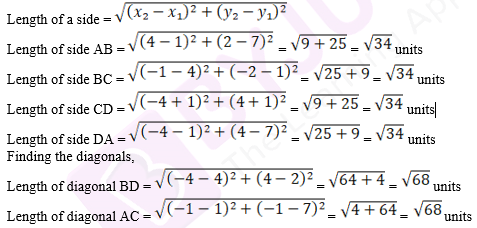As the opposite sides are equal and also the diagonals are equal the given vertices are therefore the vertices of a square.

• Hence Proved

9. Prove that the points (3, 0), (6, 4) and (- 1, 3) are vertices of a right-angled isosceles triangle.

Solution:

Let the vertices of the triangle ABC be: A(3, 0), B(6, 4) and C (- 1, 3)

We know that,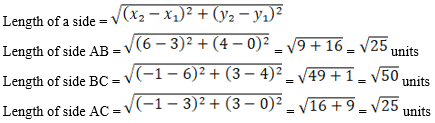It’s seen that AB = AC, Thus, it’s an isosceles triangle.

Verifying the Pythagoras theorem, we have

BC2 = AB2 + AC2

(√50)2 = (√25)2 + (√25)2

50 = 25 + 25

50 = 50

As BC2 = AB2 + AC2

Therefore, the given vertices are of a right-angled isosceles triangle.

• Hence Proved

10. Prove that (2, -2), (-2, 1) and (5, 2) are the vertices of a right angled triangle. Find the area of the triangle and the length of the hypotenuse.

Solution:

From given,

Let consider the vertices of a triangle ABC as: A(2, -2), B(-2, 1) and C(5, 2)

We know that,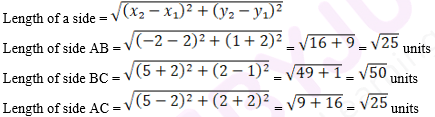It’s seen that AB = AC, thus the triangle is an isosceles triangle.

Verifying the Pythagoras theorem, we have

BC2 = AB2 + AC2

(√50)2 = (√25)2 + (√25)2

50 = 25 + 25

50 = 50

As BC2 = AB2 + AC2

Therefore, the given triangle is right angled triangle.

Now,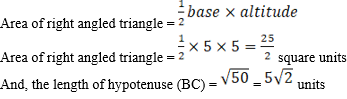• Hence Proved

11. Prove that the points (2a, 4a), (2a, 6a) and (2a + √3a, 5a) are the vertices of an equilateral triangle.

Solution:

From given,

Let’s consider the vertices of a triangle ABC as: A(2 a, 4 a), B(2 a, 6 a) and C(2a + √3a, 5a)

We know that,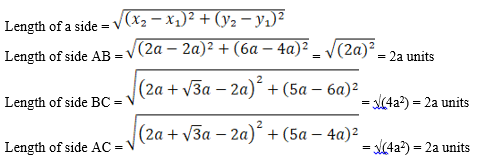As all the sides are equal the triangle is an equilateral triangle.

Thus, the given vertices are of an equilateral triangle.

• Hence Proved

12. Prove that the points (2, 3), (-4, -6) and (1, 3/2) do not form a triangle.

Solution:

From given,

Let’s consider the vertices of a triangle ABC as: A(2, 3), B(-4, -6) and C(1, 3/2)

We know that,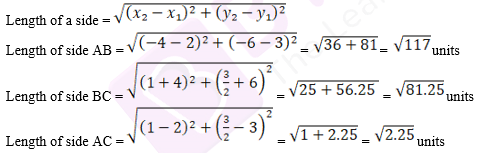Thus, the given vertices do not form a triangle as the sum of two sides of a triangle is not greater than third side.

• Hence Proved

13. The points A (2, 9), B (a, 5) and C (5, 5) are the vertices of a triangle ABC right triangle ABC right angled at B. Find the values of a and hence the area of triangle ABC.

Solution:

Given,

A right triangle ABC, right angled at B.

Points A (2, 9), B (a, 5) and C (5, 5)

So, AC is the hypotenuse

Thus, from Pythagoras theorem we have

AC2 = AB2 + BC2

[(5 – 2)2 + (5 – 9)2] = [(a – 2)2 + (5 – 9)2] + [(5 – a)2 + (5 – 5)2] [32 + (-4)2] = [(a – 2)2 + (-4)2] + [(5 – a)2 + 0]

9 + 16 = a2 – 4a + 4 + 16 + 25 – 10a + a2

2a2 – 14a + 20 = 0

a2 – 7a + 10 = 0

(a – 5)(a – 2) = 0 [By factorization method]

So,

a = 5 or 2

Here, a = 5 is not possible as it coincides with point C. So, for a triangle to form the value of a = 2 is correct.

Thus, the coordinates of point B is (2, 5).

Now, the area of triangle ABC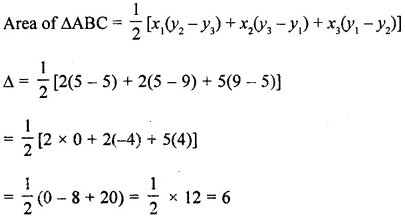Therefore, the area of triangle ABC is 6 sq. units

14. Show that the quadrilateral whose vertices are (2, -1), (3, 4), (-2, 3) and (-3, -2) is a rhombus.

Solution:

Let A(2, -1), B(3 ,4), C(-2, 3) and D(-3, -2)

Then we have,

Length of AB = √[(3 – 2)2 + (4 – (-1))2] = √[(1)2 + (5)2] = √[1 + 25] = √26 units

Length of BC = √[(3 – (-2))2 + (4 – 3)2] = √[(5)2 + (1)2] = √[25 + 1] = √26 units

Length of CD = √[(-2 – (-3))2 + (3 – 2)2] = √[(-5)2 + (1)2] = √[25 + 1] = √26 units

Length of AD = √[(-3 – 2)2 + (-2 – (-1))2] = √[(-5)2 + (-1)2] = √[25 + 1] = √26 units

As AB = BC = CD = AD

We can say that,

15. Two vertices of an isosceles triangle are (2, 0) and (2, 5). Find the third vertex if the length of the equal sides is 3.

Solution:

Let the third vertex be C (x, y)

And, given A (2, 0) & B (2, 5)

We have,

Length of AB = √[(2 – 2)2 + (5 – 0)2] = √[(0)2 + (5)2] = √[0 + 25] = 5 units

Length of BC = √[(x – 2)2 + (y – 5)2] = √[x2 – 4x + 4 + y2 – 10y + 25]

= √[ x2 – 4x + y2 – 10y + 29] units

Length of AC = √[(x – 2)2 + (y – 0)2] = √[x2 – 4x + 4 + y2] units

Given that,

AC = BC = 3

So, AC2 = BC2 = 9

x2 – 4x + 4 + y2 = x2 – 4x + y2 – 10y + 29

10y = 25

y = 25/10 = 2.5

And,

AC2 = 9

x2 – 4x + 4 + y2 = 9

x2 – 4x + 4 + (2.5)2 = 9

x2 – 4x + 4 + 6.25 = 9

x2 – 4x + 1.25 = 0

D = (-4)2 – 4 x 1 x 1.25 = 16 – 5 = 11

So, the roots are

x = -(-4) + √11/ 2 = (4 + 3.31)/ 2 = 3.65

And,

x = -(-4) – √11/ 2 = (4 – 3.31)/ 2 = 0.35

Therefore, the third vertex can be C (3.65, 2.5) or (0.35, 2.5)

16. Which point on x – axis is equidistant from (5, 9) and (-4, 6)?

Solution:

Let A (5, 9) and B (-4, 6) be the given points

Let the point on x – axis equidistant from the above points be C(x, 0)

Now, we have

AC = √[(x – 5)2 + (0 – 9)2] = √[x2 – 10x + 25 + 81] = √[x2 – 10x + 106]

And,

BC = √[(x – (-4))2 + (0 – 6)2] = √[x2 + 8x + 16 + 36] = √[x2 + 8x + 52]

As AC = BC (given condition)

So, AC2 = BC2

x2 – 10x + 106 = x2 + 8x + 52

18x = 54

x = 3

Therefore, the point on the x-axis is (3, 0)

17. Prove that the points (-2, 5), (0, 1) and (2, -3) are collinear.

Solution:

Let A (-2, 5), B(0, 1) and C (2, -3) be the given points

So, we have

AB = √[(0 – (-2))2 + (1 – 5)2] = √[(2)2 + (-4)2] = √[4 + 16] = √20 = 2√5 units

BC = √[(2 – 0)2 + (-3 – 1)2] = √[(2)2 + (-4)2] = √[4 + 16] = √20 = 2√5 units

AC = √[(2 – (-2))2 + (-3 – 5)2] = √[(4)2 + (-8)2] = √[16 + 64] = √80 = 4√5 units

Now, it’s seen that

AB + BC = AC

2√5 + 2√5 = 4√5

4√5 = 4√5

Therefore, we can conclude that the given points (-2, 5), (0, 1) and (2, -3) are collinear

18. The coordinates of the point P are (-3, 2). Find the coordinates of the point Q which lies on the line joining P and origin such that OP = OQ.

Solution: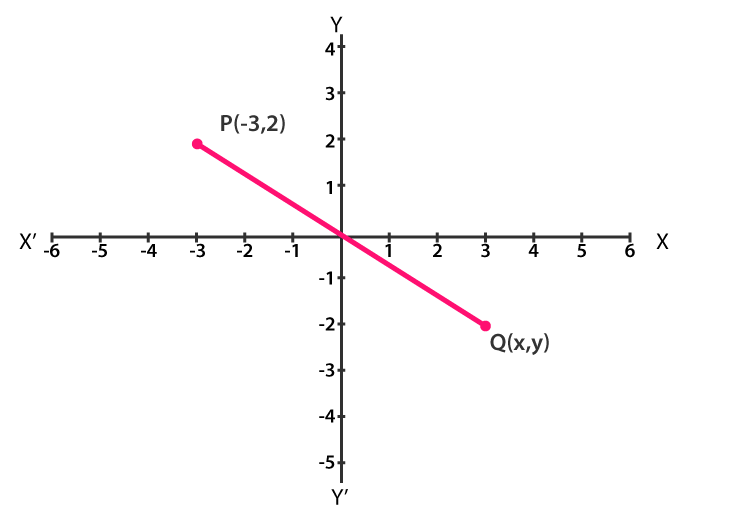Let the coordinates of Q be taken as (x, y)

As Q lies on the line joining P and O(origin) with OP = OQ

Then, by mid-point theorem

(x – 3)/2 = 0

And,

(y + 2)/ 2 = 0

∴ x = 3, y = -2

Therefore, the coordinates of point Q are (3, -2)

19. Which point on the y-axis is equidistant from (2, 3) and (-4, 1)?

Solution:

Let A (2, 3) and B (-4, 1) be the given points

Let the point on y – axis equidistant from the above points be C (0, y)

Now, we have

AC = √[(0 – 2)2 + (y – 3)2] = √[y2 – 6y + 9 + 4] = √[y2 – 6y + 13]

And,

BC = √[(0 – (-4))2 + (y – 1)2] = √y2 – 2y + 1 + 16] = √[y2 – 2y + 17]

As AC = BC (given condition)

So, AC2 = BC2

y2 – 6y + 13 = y2 – 2y + 17

-4y = 4

y = -1

Therefore, the point on the y-axis is (0, -1)

20. The three vertices of a parallelogram are (3, 4), (3, 8) and (9, 8). Find the fourth vertex.

Solution: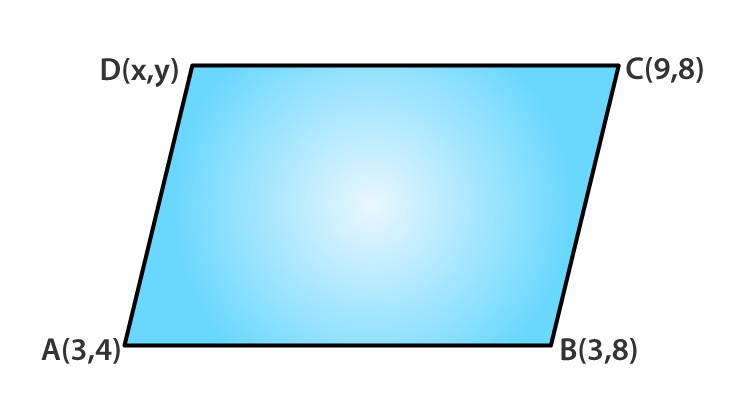Let A (3, 4), B (3, 8) and C (9, 8) be the given points.

And let the fourth vertex be D(x, y)

We know that,

In a parallelogram the diagonal bisect each other.

So, the mid-point of AC should be the same as the mid-point of BC

By mid-point theorem,

Mid-point of AC = (3 + 9/ 2), (4 + 8/ 2) = (6, 6)

Now,

The mid-point of BD = (3 + x/ 2, 8 + y/ 2)

And this point must be equal to (6, 6)

So, we have

(3 + x)/ 2 = 6 (8 + y)/ 2 = 6

3 + x = 12 8 + y = 12

x = 9 y = 4

Therefore, the fourth vertex is D (9, 4)

21. Find a point which is equidistant from the points A (-5, 4) and B (-1, 6). How many such points are there?

Solution:

Let P(x, y) be the equidistant point from points A (-5, 4) and B (-1, 6).

So, the mid-point can be the required point

(x, y) = ( (-5 – 1/ 2), (4 + 6)/2 )

(x , y) = (-6/2 , 10/2) = (-3, 5)

Thus, the required point is (-3, 5)

Now,

We also know that, AP = BP

So, AP2 = BP2

(x + 5)2 + (y – 4)2 = (x + 1)2 + (y – 6)2

x2 + 25 + 10 + y2 – 8y + 16 = x2 + 2x + 1 + y2 – 12y + 36

10x + 41 – 8y = 2x + 37 – 12y

8x + 4y + 4 = 0

2x + y + 1 = 0

Therefore, all the points which lie on the line 2x + y + 1 = 0 are equidistant from A and B.

22. The center of a circle is (2a, a – 7). Find the values of a if the circle passes through the point (11, -9) and has diameter 10√2 units.

Solution:

Given,

Diameter of the circle = 10√2 units

So, the radius = 5√2 units

Let the center of a circle be 0(2a, a-7) and the circle passes though the point P (11, -9).

Then, OP is the radius of the circle

OP = 5√2

OP2 = (5√2) = 50

(11- 2a)2 + (-9 – a + 7)2 = 50

121 – 44a + 4a2 + 4 + a2 + 4a = 50

5a2 – 40a + 75 = 0

a2 – 8a + 15 = 0

(a – 5)(a – 3) = 0 [Factorisation method]

So, a = 5 or a = 3

23. Ayush starts walking from his house to office, Instead of going to the office directly, he goes to bank first, from there to his daughter’s school and then reaches the office. What is the extra distance travelled by Ayush in reaching the office? (Assume that all distance covered are in straight lines). If the house is situated at (2, 4), bank at (5, 8) school at (13, 14) and office at (13, 26) and coordinates are in kilometer.

Solution:

The position of Ayush’s house is (2, 4) and the position of the bank is (5, 8).

So, the distance between the house and the bank,

d1 = √[(5 – 2)2 + (8 – 4)2] = √[(3)2 + (4)2] = √[9 + 16] = √25 = 5 km

The position of the bank is (5, 8) and the position of the school is (13, 14).

So, the distance between the bank and the school,

d2 = √[(13 – 5)2 + (14 – 8)2] = √[(8)2 + (6)2] = √[64 + 36] = √100 = 10 km

The position of the school is (13, 14) and the position of the office is (13, 26).

So, the distance between the school and the office,

d3 = √[(13 – 13)2 + (26 – 14)2] = √[(0)2 + (12)2] = √144 = 12 km

Let d be the total distance covered by Ayush

d = d1 + d2 + d3 = 5 + 10 + 12 = 27 km

Let the D be the shortest distance from Ayush’s house to the office,

D = √[(13 – 2)2 + (26 – 4)2] = √[(11)2 + (22)2] = √[121+ 484] = √605 = 24.6 km

Thus, the extra distance covered by Ayush = d – D = 27 – 24.6 = 2.4 km

24. Find the value of k, if the point P(0, 2) is equidistant from (3, k) and (k, 5).

Solution:

Let the point P (0, 2) is equidistant from A (3, k) and B (k, 5)

So, PA = PB

PA2 = PB2

(3 -0)2 + (k -2)2 = (k – 0)2 + (5 – 2)2

9 + k2 + 4 – 4k – k2 – 9 = 0

4 – 4k = 0

-4k = -4

Therefore, the value of k = 1

25. If (-4, 3) and (4, 3) are two vertices of an equilateral triangle, find the coordinates of the third vertex, given that the origin lies in the (i) interior (ii) exterior of the triangle.

Solution:

Let B (-4, 3) and C (4, 3) be the given two vertices of the equilateral triangle.

Let A (x, y) be the third vertex.

Then, we have

AB = BC = AC

Let us consider the part AB = BC

AB2 = BC2

(-4 – x)2 + (3 – y)2 = (4 + 4)2 + (3 – 3)2

16 + x2 + 8x + 9 + y2 – 6y = 64

x2 + y2 + 8x – 6y = 39

Now, let us consider AB = AC

AB2 = AC2

(-4 – x)2 + (3 – y)2 = (4 – x)2 + (3 – y)2

16 + x2 + 8x + 9 + y2 – 18y = 16 + x2 – 8x + 9 + y2 – 6y

16x = 0

x = 0

Now, BC = AC

BC2 = AC2

(4 + 4)2 + (3 – 3)2 = (4 – 0)2 + (3 – y)2

64 + 0 = 16 + 9 + y– 6y

64 = 16 + (3 – y)2

(3 – y)2 = 48

3 – y = ± 4√3

y = 3 ± 4√3

Therefore, the coordinates of the third vertex

(i) When origin lies in the interior of the triangle is (0, 3 – 4√3)

(ii) When origin lies in the exterior of the triangle is (0, 3 + 4√3)

26. Show that the points (-3, 2), (-5, -5), (2, -3) and (4, 4) are the vertices of a rhombus. Find the area of this rhombus.

Solution: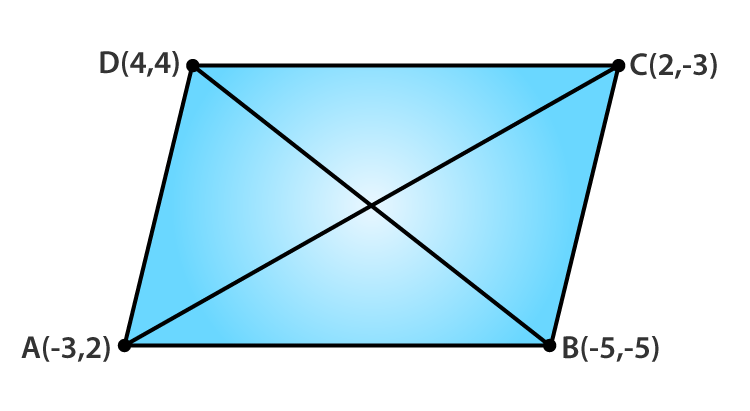Let A(-3, 2), B(-5, -5), C(2, -3) and D(4, 4) be the given points.

Then we have,

AB = √[(-5 + 3)2 + (-5 – 2)2] = √[(2)2 + (7)2] = √[4 + 49] = √53 units

BC = √[(2 + 5)2 + (-3 + 5)2] = √[(7)2 + (2)2] = √[49 + 4] = √53 units

CD = √[(4 – 2)2 + (4 + 3)2] = √[(2)2 + (7)2] = √[4 + 49] = √53 units

AD = √[(4 + 3)2 + (4 – 2)2] = √[(7)2 + (2)2] = √[49 + 4] = √53 units

And the diagonals,

AC = √[(2 + 3)2 + (-3 – 2)2] = √[(5)2 + (-5)2] = √[25 + 25] = 5√2 units

BD = √[(4 + 5)2 + (4 + 5)2] = √[(9)2 + (9)2] = √[81 + 81] = 9√2 units

It’s seen that,

As AB = BC = CD = AD and the diagonals AC ≠ BD

ABCD is a rhombus.

Now,

Area of rhombus ABCD = ½ x AC x BD = ½ x 5√2 x 9√2 = 45 sq. units

27. Find the coordinates of the circumcenter of the triangle whose vertices are (3, 0), (-1, -6) and (4, -1). Also find the circumradius.

Solution: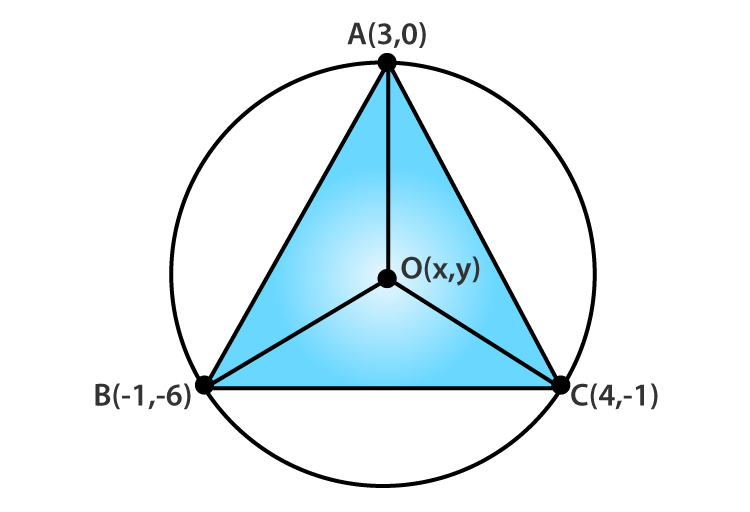Let A(3, 0), B(-1, -6) and C(4 , -1) be the given points.

Let O(x , y) be the circumcenter of the triangle

Then, OA = OB = OC

OA2 = OB2

(x – 3)2 + (y – 0)2 = (x + 1)2 + (y + 6)2

x2 + 9 – 6x + y2 = x2 + 1 + 2x + y2 + 36 + 12y

-8x -12y = 28

2x + 3y = -7 …..(i) [After simplification]

Again,

OB2 = OC2

(x + 1)2 + (y + 6)2 = (x – 4)2 + (y + 1)2

x2 + 2x + 1 + y2 + 36 + 12y = x2 + 16 – 8x + y2 + 1 + 2y

10x + 10y = -20

x + y = -2 …..(ii) [After simplification]

Hence, the circumcenter of the triangle is (1, -3)

Circumradius = distance from any of the given points (say B)

=√[(1 + 1)2 + (-3 + 6)2] = √(4 + 9)

= √13 units

28. Find a point on the x-axis which is equidistant from the points (7, 6) and (-3, 4).

Solution:

Let A(7, 6) and B(-3, 4) be the given points.

Let P(x, 0) be the point on the x-axis such that PA = PB

So, PA2 = PB2

(x – 7)2 + (0 – 6)2 = (x + 3)2 + (0 – 4)2

x2 + 49 – 14x + 36 = x2 + 9 + 6x + 16

-20x = -60

x = 3

Therefore, the point on x-axis is (3, 0).